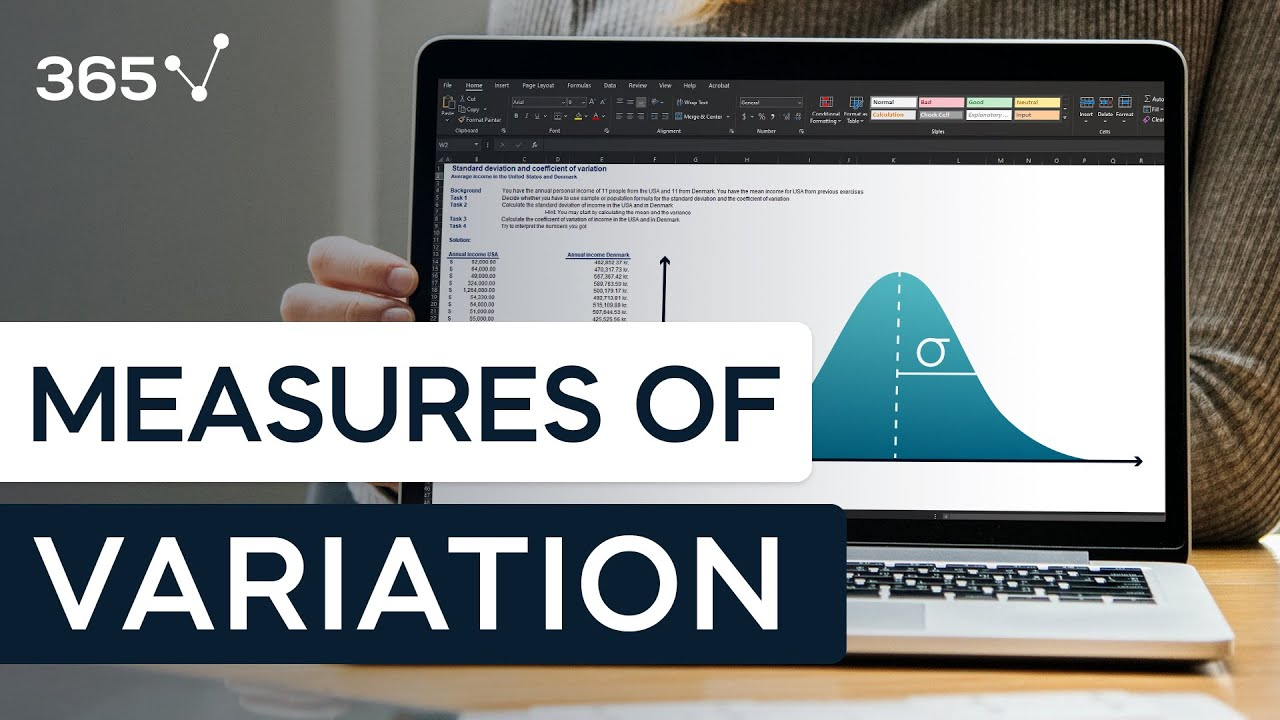Variance, Standard Deviation, Coefficient of Variation
The coefficient of variation, variance, and standard deviation are the most widely used measures of variability. We'll discuss each of these in turn, finishing off with the coefficient of variation. ✅Get 20% OFF the data science training! http://bit.ly/32ik46v
Variance measures the dispersion of a set of data points around their mean value. Population variance, denoted by sigma squared, is equal to the sum of squared differences between the observed values and the population mean, divided by the total number of observations.
Sample variance, on the other hand, is denoted by s squared and is equal to the sum of squared differences between observed sample values and the sample mean, divided by the number of sample observations minus 1.
✅http://bit.ly/2v15NPp
While variance is a common measure of data dispersion, in most cases the figure you will obtain is pretty large and hard to compare as the unit of measurement is squared. The easy fix is to calculate its square root and obtain a statistic known as standard deviation. In most analyses you perform, standard deviation will be much more meaningful than variance.
Alright. The other measure we still have to introduce is the coefficient of variation. It is equal to the standard deviation, divided by the mean. Another name for the term is relative standard deviation. This is an easy way to remember its formula – it is simply the standard deviation relative to the mean. As you probably guessed, there is a population and sample formula once again.
Connect with us on our social media platforms:
✅Website: https://bit.ly/2TrLiXb
✅Medium: https://medium.com/@365datascience
✅Pinterest: https://www.pinterest.com/365datascience/
✅Reddit: https://www.reddit.com/user/365datascience
✅Tumblr: https://www.tumblr.com/blog/365datascienceblog
✅Instagram: https://www.instagram.com/365datascience
✅Q&A Hub: https://365datascience.com/qa-hub/
??Prepare yourself for a career in data science with our comprehensive program??
✅http://bit.ly/32ik46v
Get in touch about the training at:
support@365datascience.com
Comment, like, share, and subscribe! We will be happy to hear from you and will get back to you!
#coefficientofvariation #statistics #variance #standarddeviation365 Data Science
At 365 Data Science, we all come to work every day because we want to solve the biggest problem in data science. Education. People who want to enter the field do not know where to start. They wonder whether they need a PhD, or perhaps a few years in a...